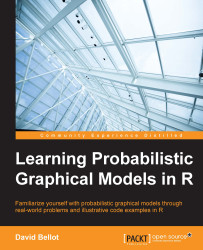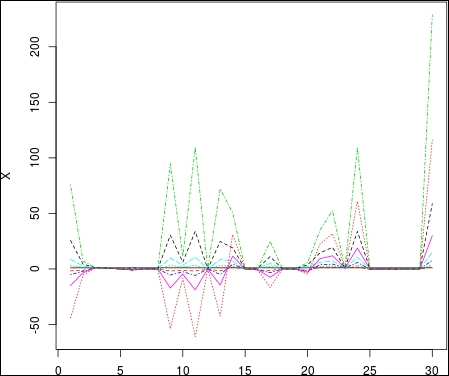•#### Learning Probabilistic Graphical Models in R#### Overview of this book

Probabilistic graphical models (PGM, also known as graphical models) are a marriage between probability theory and graph theory. Generally, PGMs use a graph-based representation. Two branches of graphical representations of distributions are commonly used, namely Bayesian networks and Markov networks. R has many packages to implement graphical models. We’ll start by showing you how to transform a classical statistical model into a modern PGM and then look at how to do exact inference in graphical models. Proceeding, we’ll introduce you to many modern R packages that will help you to perform inference on the models. We will then run a Bayesian linear regression and you’ll see the advantage of going probabilistic when you want to do prediction. Next, you’ll master using R packages and implementing its techniques. Finally, you’ll be presented with machine learning applications that have a direct impact in many fields. Here, we’ll cover clustering and the discovery of hidden information in big data, as well as two important methods, PCA and ICA, to reduce the size of big problems.
Learning Probabilistic Graphical Models in RCreditswww.PacktPub.comPrefaceFree Chapter
Probabilistic ReasoningExact InferenceLearning ParametersBayesian Modeling – Basic ModelsApproximate InferenceBayesian Modeling – Linear ModelsProbabilistic Mixture ModelsAppendixIndex## Bayesian linear models

In this section, we are going to extend the standard linear regression model using the Bayesian paradigm. One of the goals is to put prior knowledge on the parameters of the models to help to solve the over-fitting problem.

### Over-fitting a model

One immense benefit of going Bayesian when doing a linear model is to have better control of the parameters. Let's do an initial experiment to see what happens when the parameters are completely out of control.

We are going to generate a simple model in R and look at the parameters when they are fitted with the standard approach for linear models.

Let's first generate some data points at random to obtain 10 variables and plot them:

```N <- 30
x <- runif(N, -2, 2)
X <- cbind(rep(1, N), x, x^2, x^3, x^4, x^5, x^6, x^7, x^8)
matplot(X, t='l')```Next we generate the dependent variable following the model:

y = Xβ + ϵ

Here, ϵ is a Gaussian noise of variance σ2. We use the following code in R and plot the variable y. As we use randomly...